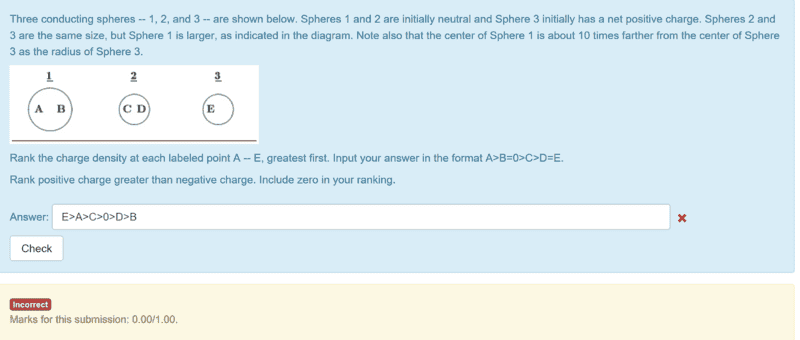# Rank charges in spheres (Physics 2)

## Homework Statement## Homework Equations

None (conceptual)

## The Attempt at a Solution

My logic here is this, Sphere 3 has a net positive charge so it is repelling the positives in sphere 2 and attracting the negatives in sphere 2. This means that D has negative charge and C has positive charge. Since sphere 1 and sphere 2 are both neutral to start they have no effect on eachother so the primary catalyst here is sphere 3. after sphere 3 rearranges the electrons on sphere 2 sphere 2 has an effect on sphere 1, attracting the negatives towards it and repelling the positives. That is how I got my ranking here. Am I correct with my thinking here?